# 1.5 Exponential and logarithmic functions  (Page 7/17)

 Page 7 / 17

## Evaluating hyperbolic functions

1. Simplify $\text{sinh}\left(5\phantom{\rule{0.1em}{0ex}}\text{ln}\phantom{\rule{0.1em}{0ex}}x\right).$
2. If $\text{sinh}\phantom{\rule{0.1em}{0ex}}x=3\text{/}4,$ find the values of the remaining five hyperbolic functions.
1. Using the definition of the $\text{sinh}$ function, we write
$\text{sinh}\left(5\phantom{\rule{0.1em}{0ex}}\text{ln}\phantom{\rule{0.1em}{0ex}}x\right)=\frac{{e}^{5\phantom{\rule{0.1em}{0ex}}\text{ln}\phantom{\rule{0.1em}{0ex}}x}-{e}^{-5\phantom{\rule{0.1em}{0ex}}\text{ln}\phantom{\rule{0.1em}{0ex}}x}}{2}=\frac{{e}^{\text{ln}\left({x}^{5}\right)}-{e}^{\text{ln}\left({x}^{-5}\right)}}{2}=\frac{{x}^{5}-{x}^{-5}}{2}.$
2. Using the identity ${\text{cosh}}^{2}x-{\text{sinh}}^{2}x=1,$ we see that
${\text{cosh}}^{2}x=1+{\left(\frac{3}{4}\right)}^{2}=\frac{25}{16}.$

Since $\text{cosh}\phantom{\rule{0.1em}{0ex}}x\ge 1$ for all $x,$ we must have $\text{cosh}\phantom{\rule{0.1em}{0ex}}x=5\text{/}4.$ Then, using the definitions for the other hyperbolic functions, we conclude that $\text{tanh}\phantom{\rule{0.1em}{0ex}}x=3\text{/}5,\text{csch}\phantom{\rule{0.1em}{0ex}}x=4\text{/}3,\text{sech}\phantom{\rule{0.1em}{0ex}}x=4\text{/}5,$ and $\text{coth}\phantom{\rule{0.1em}{0ex}}x=5\text{/}3.$

Simplify $\text{cosh}\left(2\phantom{\rule{0.1em}{0ex}}\text{ln}\phantom{\rule{0.1em}{0ex}}x\right).$

$\left({x}^{2}+{x}^{-2}\right)\text{/}2$

## Inverse hyperbolic functions

From the graphs of the hyperbolic functions, we see that all of them are one-to-one except $\text{cosh}\phantom{\rule{0.1em}{0ex}}x$ and $\text{sech}\phantom{\rule{0.1em}{0ex}}x.$ If we restrict the domains of these two functions to the interval $\left[0,\infty \right),$ then all the hyperbolic functions are one-to-one, and we can define the inverse hyperbolic functions    . Since the hyperbolic functions themselves involve exponential functions, the inverse hyperbolic functions involve logarithmic functions.

## Definition

Inverse Hyperbolic Functions

$\begin{array}{cccc}{\text{sinh}}^{-1}x=\text{arcsinh}\phantom{\rule{0.1em}{0ex}}x=\text{ln}\left(x+\sqrt{{x}^{2}+1}\right)\hfill & & & {\text{cosh}}^{-1}x=\text{arccosh}\phantom{\rule{0.1em}{0ex}}x=\text{ln}\left(x+\sqrt{{x}^{2}-1}\right)\hfill \\ {\text{tanh}}^{-1}x=\text{arctanh}\phantom{\rule{0.1em}{0ex}}x=\frac{1}{2}\text{ln}\left(\frac{1+x}{1-x}\right)\hfill & & & {\text{coth}}^{-1}x=\text{arccot}\phantom{\rule{0.1em}{0ex}}x=\frac{1}{2}\text{ln}\left(\frac{x+1}{x-1}\right)\hfill \\ {\text{sech}}^{-1}x=\text{arcsech}\phantom{\rule{0.1em}{0ex}}x=\text{ln}\left(\frac{1+\sqrt{1-{x}^{2}}}{x}\right)\hfill & & & {\text{csch}}^{-1}x=\text{arccsch}\phantom{\rule{0.1em}{0ex}}x=\text{ln}\left(\frac{1}{x}+\frac{\sqrt{1+{x}^{2}}}{|x|}\right)\hfill \end{array}$

Let’s look at how to derive the first equation. The others follow similarly. Suppose $y={\text{sinh}}^{-1}x.$ Then, $x=\text{sinh}\phantom{\rule{0.1em}{0ex}}y$ and, by the definition of the hyperbolic sine function, $x=\frac{{e}^{y}-{e}^{\text{−}y}}{2}.$ Therefore,

${e}^{y}-2x-{e}^{\text{−}y}=0.$

Multiplying this equation by ${e}^{y},$ we obtain

${e}^{2y}-2x{e}^{y}-1=0.$

This can be solved like a quadratic equation, with the solution

${e}^{y}=\frac{2x±\sqrt{4{x}^{2}+4}}{2}=x±\sqrt{{x}^{2}+1}.$

Since ${e}^{y}>0,$ the only solution is the one with the positive sign. Applying the natural logarithm to both sides of the equation, we conclude that

$y=\text{ln}\left(x+\sqrt{{x}^{2}+1}\right).$

## Evaluating inverse hyperbolic functions

Evaluate each of the following expressions.

${\text{sinh}}^{-1}\left(2\right)$
${\text{tanh}}^{-1}\left(1\text{/}4\right)$

${\text{sinh}}^{-1}\left(2\right)=\text{ln}\left(2+\sqrt{{2}^{2}+1}\right)=\text{ln}\left(2+\sqrt{5}\right)\approx 1.4436$

${\text{tanh}}^{-1}\left(1\text{/}4\right)=\frac{1}{2}\text{ln}\left(\frac{1+1\text{/}4}{1-1\text{/}4}\right)=\frac{1}{2}\text{ln}\left(\frac{5\text{/}4}{3\text{/}4}\right)=\frac{1}{2}\text{ln}\left(\frac{5}{3}\right)\approx 0.2554$

Evaluate ${\text{tanh}}^{-1}\left(1\text{/}2\right).$

$\frac{1}{2}\text{ln}\left(3\right)\approx 0.5493.$

## Key concepts

• The exponential function $y={b}^{x}$ is increasing if $b>1$ and decreasing if $0 Its domain is $\left(\text{−}\infty ,\infty \right)$ and its range is $\left(0,\infty \right).$
• The logarithmic function $y={\text{log}}_{b}\left(x\right)$ is the inverse of $y={b}^{x}.$ Its domain is $\left(0,\infty \right)$ and its range is $\left(\text{−}\infty ,\infty \right).$
• The natural exponential function is $y={e}^{x}$ and the natural logarithmic function is $y=\text{ln}\phantom{\rule{0.1em}{0ex}}x={\text{log}}_{e}x.$
• Given an exponential function or logarithmic function in base $a,$ we can make a change of base to convert this function to any base $b>0,b\ne 1.$ We typically convert to base $e.$
• The hyperbolic functions involve combinations of the exponential functions ${e}^{x}$ and ${e}^{\text{−}x}.$ As a result, the inverse hyperbolic functions involve the natural logarithm.

For the following exercises, evaluate the given exponential functions as indicated, accurate to two significant digits after the decimal.

$f\left(x\right)={5}^{x}$ a. $x=3$ b. $x=\frac{1}{2}$ c. $x=\sqrt{2}$

a. 125 b. 2.24 c. 9.74

$f\left(x\right)={\left(0.3\right)}^{x}$ a. $x=-1$ b. $x=4$ c. $x=-1.5$

$f\left(x\right)={10}^{x}$ a. $x=-2$ b. $x=4$ c. $x=\frac{5}{3}$

a. 0.01 b. 10,000 c. 46.42

$f\left(x\right)={e}^{x}$ a. $x=2$ b. $x=-3.2$ c. $x=\pi$

For the following exercises, match the exponential equation to the correct graph.

1. $y={4}^{\text{−}x}$
2. $y={3}^{x-1}$
3. $y={2}^{x+1}$
4. $y={\left(\frac{1}{2}\right)}^{x}+4$
5. $y=\text{−}{3}^{\text{−}x}$
6. $y=1-{5}^{x}$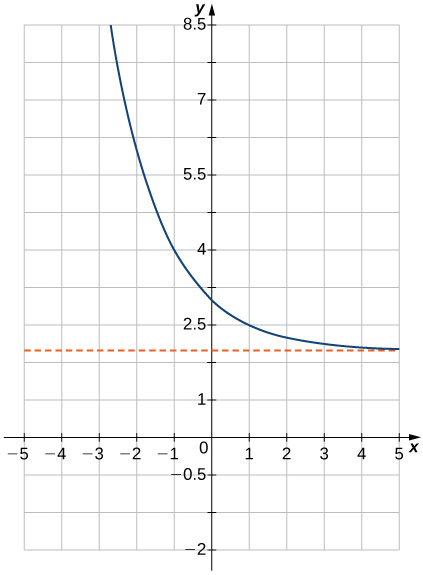d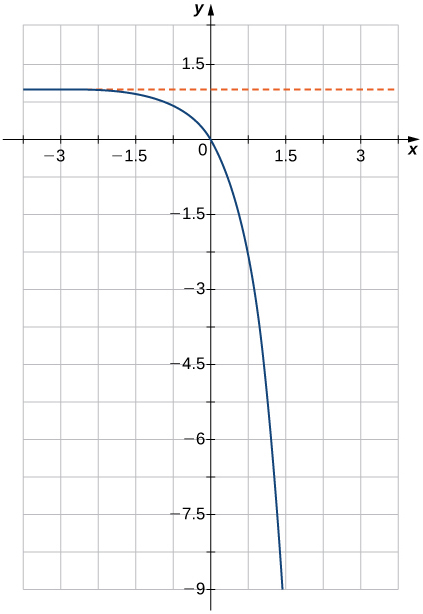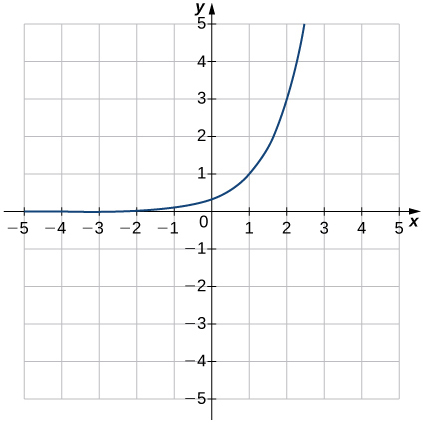b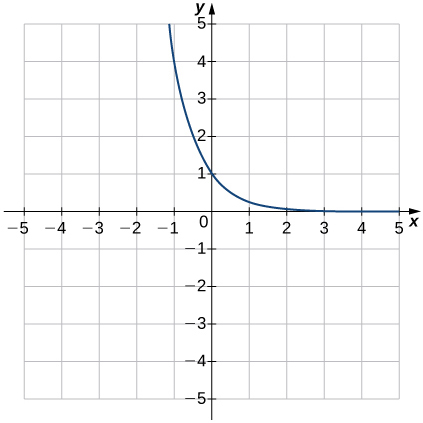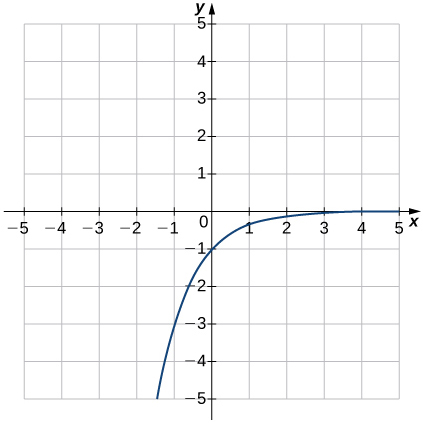e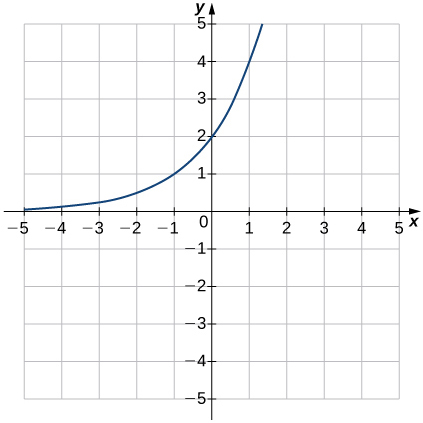For the following exercises, sketch the graph of the exponential function. Determine the domain, range, and horizontal asymptote.

f(x) = x-2 g(x) = 3x + 5 fog(x)? f(x)/g(x)
fog(x)= f(g(x)) = x-2 = 3x+5-2 = 3x+3 f(x)/g(x)= x-2/3x+5
diron
pweding paturo nsa calculus?
jimmy
how to use fundamental theorem to solve exponential
find the bounded area of the parabola y^2=4x and y=16x
what is absolute value means?
Chicken nuggets
Hugh
🐔
MM
🐔🦃 nuggets
MM
(mathematics) For a complex number a+bi, the principal square root of the sum of the squares of its real and imaginary parts, √a2+b2 . Denoted by | |. The absolute value |x| of a real number x is √x2 , which is equal to x if x is non-negative, and −x if x is negative.
Ismael
find integration of loge x
find the volume of a solid about the y-axis, x=0, x=1, y=0, y=7+x^3
how does this work
Can calculus give the answers as same as other methods give in basic classes while solving the numericals?
log tan (x/4+x/2)
Rohan
Rohan
y=(x^2 + 3x).(eipix)
Claudia
Ismael
A Function F(X)=Sinx+cosx is odd or even?
neither
David
Neither
Lovuyiso
f(x)=1/1+x^2 |=[-3,1]
apa itu?
fauzi
determine the area of the region enclosed by x²+y=1,2x-y+4=0
Hi
MP
Hi too
Vic
hello please anyone with calculus PDF should share
Which kind of pdf do you want bro?
Aftab
hi
Abdul
can I get calculus in pdf
Abdul
explain for me
Usman
How to use it to slove fraction
Hello please can someone tell me the meaning of this group all about, yes I know is calculus group but yet nothing is showing up
Shodipo
You have downloaded the aplication Calculus Volume 1, tackling about lessons for (mostly) college freshmen, Calculus 1: Differential, and this group I think aims to let concerns and questions from students who want to clarify something about the subject. Well, this is what I guess so.
Jean
Im not in college but this will still help
nothing
how en where can u apply it
Migos
how can we scatch a parabola graph
Ok
Endalkachew
how can I solve differentiation?
with the help of different formulas and Rules. we use formulas according to given condition or according to questions
CALCULUS
For example any questions...
CALCULUS
v=(x,y) وu=(x,y ) ∂u/∂x* ∂x/∂u +∂v/∂x*∂x/∂v=1
log tan (x/4+x/2)
Rohan
what is the procedures in solving number 1?ByBy OpenStaxBy RhodesBy Janet ForresterByBy Richley CrapoBy John GabrieliBy Richley CrapoBy OpenStaxBy Madison Christian